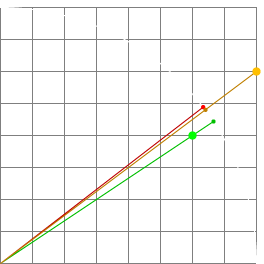# Improved method for storing normals as a series of bytes

by Michael Kwaśnicki

This article describes a method to pack vertex normals into just three bytes using an approach that provides better results than just regular conversion.

### Why do this at all

Graphics processors (GPUs) are blazing fast nowadays and they can perform a huge amount of computation. A problem arises when it comes to provide data to keep the GPU busy. You just can’t feed it as quick as it computes the output. Especially on mobile platforms the memory bandwidth is a problem. So packing the data more densely helps to reduce the pressure on the memory bandwidth and allows to do more on the GPU.

### The naïve approach

Normals are unit vectors that point away from a surface. I’m using here the C language paradigm to explain the required steps. Typically they are constructed from three floating point numbers. Each component of this vector takes values of [-1, 1] thus converting them into bytes will be horrible. With the default round towards zero behavior the components will be zero for most cases. Thus they have to be scaled with `UCHAR_MAX` = 127 (defined in `limits.h`). It is also possible to call `roundf()` to round the floating point number prior to conversion but the result is still bound to the surface of a quantized sphere.

``````float x;
byte bx = x * UCHAR_MAX;``````

That would be the straight forward approach. On the OpenGL side one needs to provide those byte normals for drawing. This can either be done by telling OpenGL to normalize the input, which converts the into float and then maps the value from [-128, 127] to [-1, 1]. (There is a change on that behavior in the latest OpenGL specification. Now it maps from [-127, 127] to [-1, 1] as the prior approach made it impossible to represent 0 exactly.) Anyway, this approach does not guarantee you to get normals of unit length as they are already shortened by the conversion. Or one tells OpenGL to just pass in the values as floats and call `normalize()` in the vertex shader to get unit length normals.

### The better approach

As calling `normalize()` gives better results, then why not use an input that is not limited to the surface of a sphere but does take advantage of the full byte space. So instead of believing that the byte representation of the normal as described above would be the best, one can pretty much find other points along the original normal in the byte cube that are much closer to the original normal even the length differs significantly. Those byte normals vary in length pretty much and cannot be used directly. So calling `normalize()` in the vertex shader is obligatory here.Illustrating the quantization of a direction (red) by the naïve round towards zero approach (green) and the new approach (yellow). The new approach comes much closer to the original direction after normalizing.

### Quality of the results

As we are not limited to the surface of a sphere, this approach certainly outperforms the naïve approach, which uses just a subset of byte combinations that are used by the better approach. As the 3D byte space has a size of 2563 there are 16777215 possible directions for normals (the null vector (0, 0, 0) is excluded here as it does not point anywhere). But not all directions are unique as (1, 0, 0) has the same direction as (127, 0, 0) along with all those in-between. There is a total of 3167541 ambiguities which leads to a total of 16777215 - 3167541 = 13609674 unique normal directions. Also the directions are not uniformly distributed. The more ambiguities a direction has, the worse the resolution. This implies that along the x-, y- and z-axis and also the diagonals with either x, y or z = 0 and the diagonals across the cube have the worst resolution.

### Quantifying the worst case

As the worst resolution is around the axis, we take a look on how far off a floating point normal can get. The furthest point would be half the way along a diagonal direction. So we compare the vectors (127, 0, 0) and (127, 1, 1). The angle between those is computed with atan(sqrt(2) / 127) and gives us 11.14 mrad or 0.638°. That means that any normal that is discretized this way differs at most by 5.568 mrad or 0.319° in its direction.

Actually this is also true for the naïve approach. But you can hardly get better there.Rendering of all possible normal directions as points on a sphere using an orthogonal projection with 6x super sampling. The naïve method (left) with relying on OpenGL's built in normalization. The naïve method (center) with calling normalize() in the vertex shader. The new approach (right).

### Drawbacks

While a regular normal occupies 12 bytes which is made up from three times the size of a float (4 bytes or 32 bits), the presented packing stores the same normal in just 3 bytes. Which is a saving factor of 4. But because of alignment requirements, it is given a penalty when transferring the normals as such. All data has to be aligned to 32 bits or 4 bytes. Therefore one has to add a padding of one byte to each normal and store it in 4 bytes instead of 3 bytes. But this last byte does not need to be unused. It can carry information for a different task in the vertex shader. Anyway this reduces our saving from factor 4 to factor 3.

### Conclusion

As the computation of those better unnormalized byte normals is much more expensive, it is primary intended for offline computation. But beyond that it offers way better results.